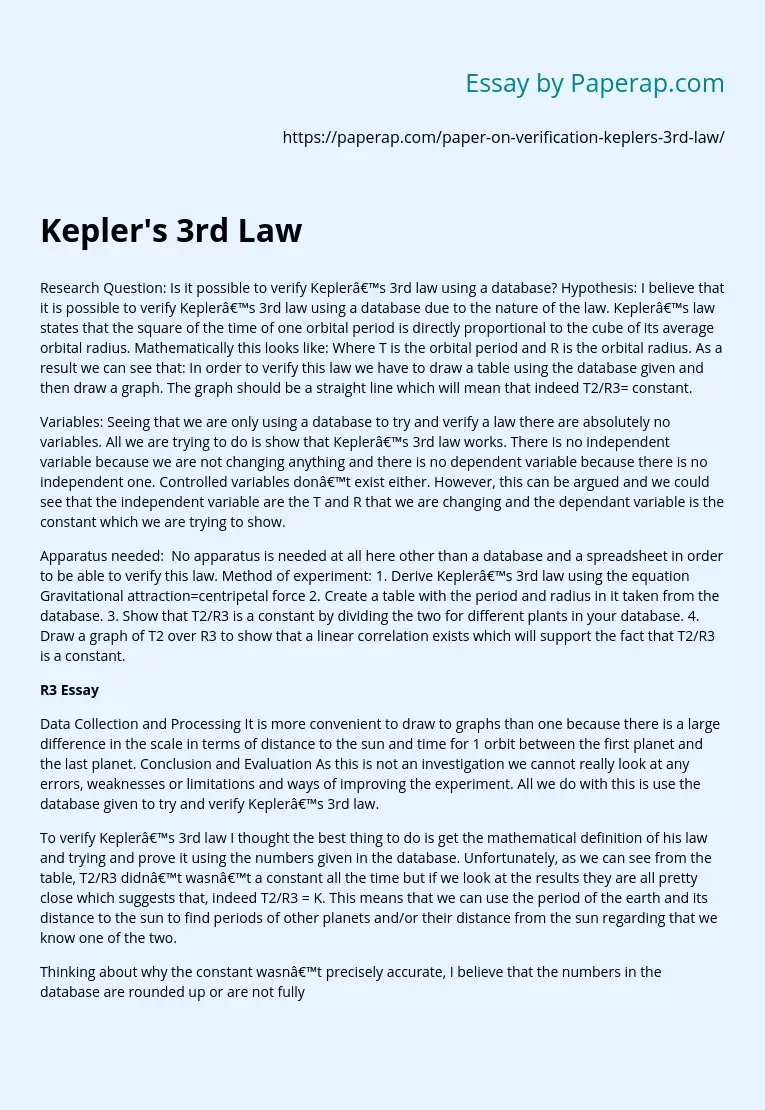# Kepler's 3rd Law

Research Question: Is it possible to verify Kepler’s 3rd law using a database? Hypothesis: I believe that it is possible to verify Kepler’s 3rd law using a database due to the nature of the law. Kepler’s law states that the square of the time of one orbital period is directly proportional to the cube of its average orbital radius. Mathematically this looks like: Where T is the orbital period and R is the orbital radius. As a result we can see that: In order to verify this law we have to draw a table using the database given and then draw a graph.

The graph should be a straight line which will mean that indeed T2/R3= constant.

Variables: Seeing that we are only using a database to try and verify a law there are absolutely no variables. All we are trying to do is show that Kepler’s 3rd law works. There is no independent variable because we are not changing anything and there is no dependent variable because there is no independent one.

Controlled variables don’t exist either. However, this can be argued and we could see that the independent variable are the T and R that we are changing and the dependant variable is the constant which we are trying to show.

Apparatus needed:  No apparatus is needed at all here other than a database and a spreadsheet in order to be able to verify this law. Method of experiment: 1.

Get quality help nowMarrie pro writerVerified

Proficient in: Experiment5 (204)

“ She followed all my directions. It was really easy to contact her and respond very fast as well. ”+84 relevant experts are online

Derive Kepler’s 3rd law using the equation Gravitational attraction=centripetal force 2. Create a table with the period and radius in it taken from the database. 3. Show that T2/R3 is a constant by dividing the two for different plants in your database. 4. Draw a graph of T2 over R3 to show that a linear correlation exists which will support the fact that T2/R3 is a constant.

## R3 Essay

Data Collection and Processing It is more convenient to draw to graphs than one because there is a large difference in the scale in terms of distance to the sun and time for 1 orbit between the first planet and the last planet. Conclusion and Evaluation As this is not an investigation we cannot really look at any errors, weaknesses or limitations and ways of improving the experiment. All we do with this is use the database given to try and verify Kepler’s 3rd law.

To verify Kepler’s 3rd law I thought the best thing to do is get the mathematical definition of his law and trying and prove it using the numbers given in the database. Unfortunately, as we can see from the table, T2/R3 didn’t wasn’t a constant all the time but if we look at the results they are all pretty close which suggests that, indeed T2/R3 = K. This means that we can use the period of the earth and its distance to the sun to find periods of other planets and/or their distance from the sun regarding that we know one of the two.

Thinking about why the constant wasn’t precisely accurate, I believe that the numbers in the database are rounded up or are not fully accurate and therefore cannot give a 100% accurate result for the constant. As a result, the graphs are also not perfect, especially the second but the fact that there is a linear correlation in the graphs is sufficient enough to verify Kepler’s law due to the fact that the numbers in the database are not 100% accurate.